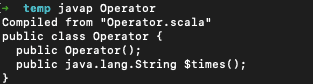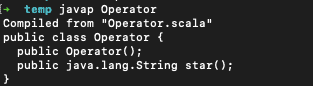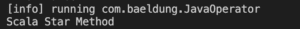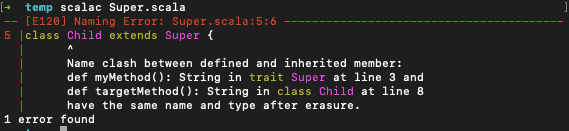## 1. Introduction

Scala 3 has introduced a lot of amazing new features to the language, such as Transparent Traits, Match Types, Extension Methods, Opaque Type Aliases, and so on.

In this tutorial, let’s look at the @targetName annotation introduced in Scala 3.

## 2. Scala Member Access Problem

Scala code is compiled into Java class files after compilation. As a result, we can invoke Scala code from Java, Kotlin, or any other JVM language. However, there are cases where a valid symbol in Scala might not be a valid symbol in Java or another JVM language.

For instance, in Scala operator overloading is supported, whereas it’s not possible in Java. As a result, the Scala compiler rewrites the overloaded function using some predefined rules. Hence, the same Scala method/symbol is not directly accessible from other languages.

Let’s look at this with an example. We can add the following code into a file Operator.scala:

``````class Operator {
def * = "Scala Star Method"
}``````

We can compile the class by using an IDE or the scalac command:

``\$ scalac Operator.scala``

If we try to access this method * from a Java class, it doesn’t compile as the Scala compiler re-writes it.

We can view the rewritten code by decompiling the class file using the javap command:

``\$ javap Operator.class``We can see that the method * is renamed as \$times by the compiler. Even though we can access the method using \$times(), it’s not really nice.

## 3. @targetName Annotation

Scala introduced the annotation @targetName in Scala 3 to solve the above usability problem. Additionally, it also helps in getting around some of the type-erasure problems.

We can use the @targetName annotation on all members except the top-level definitions.

### 3.1. Setting Alternate Name

The @targetName annotation allows us to provide an alternate name for the implementation of that definition. This provides us with a better symbol name that we can use from other JVM languages.

Let’s rewrite the Operator class with @targetName:

``````class Operator {
@targetName("star")
def * = "Scala Star Method"
}``````We can decompile the generated class again and verify the generated method name. As we can see from the above, we can now access the method from Java or another JVM language as star(). This alternate name has no bearing in Scala code and this method is accessible only as * from within any Scala files.

We can verify this by invoking this method from a Java class:

``````public class JavaOperator {
public static void main(String[] args) {
Operator op = new Operator();
System.out.println(op.star());
}
}``````

When executed, the above Java program shows the output:### 3.2. Overcoming Type Erasure

Another usage of this annotation is to get around the type erasure issue.

Let’s first look at the type-erasure issue:

``````class TypeErasure {
def sum(nums: List[Int]): Int = nums.sum
}``````

The method sum() calculates the sum of all numbers of a list.

Let’s add another method into the same class, that calculates the total number of characters in a list:

``def sum(str: List[String]): Int = str.map(_.size).sum``

However, since the type parameters in the List are erased after compilation, it causes name conflicts. We can use @targetName to avoid this conflict:

``````class TypeErasure {
def sum(nums: List[Int]): Int = nums.sum
@targetName("totalLen")
def sum(str: List[String]): Int = str.map(_.size).sum
}``````

Now the code compiles successfully even though we didn’t make any changes to the method signature. Both methods are still accessible – sum() from other Scala classes, but from other programming languages, the second method is accessible as totalLen() instead of sum().

### 3.3. Method Overriding Behavior

The @targetName annotation still needs to follow the overriding rule. That means, it can cause conflicts if there’s another method within the Scala class that has the same name as the alternate value.

Let’s get more clarity with some sample code:

``````trait Super {
def myMethod(): String = "Super"
}
class Child extends Super {
@targetName("myMethod")
def targetMethod(): String = "From Child class"
}
``````

When we compile this file, we get the following compilation error:We can fix this compilation error either by renaming the annotation value to something else or by removing the annotation altogether.

## 4. Conclusion

In this article, we looked at the @targetName annotation introduced in Scala 3. We discussed how we can use @targetName to make Scala methods accessible from other JVM languages in case of operator overloading. We also looked at how we can overcome type erasure problems in Scala using the same annotation.

As always, the code used in this tutorial is available over on GitHub.